Question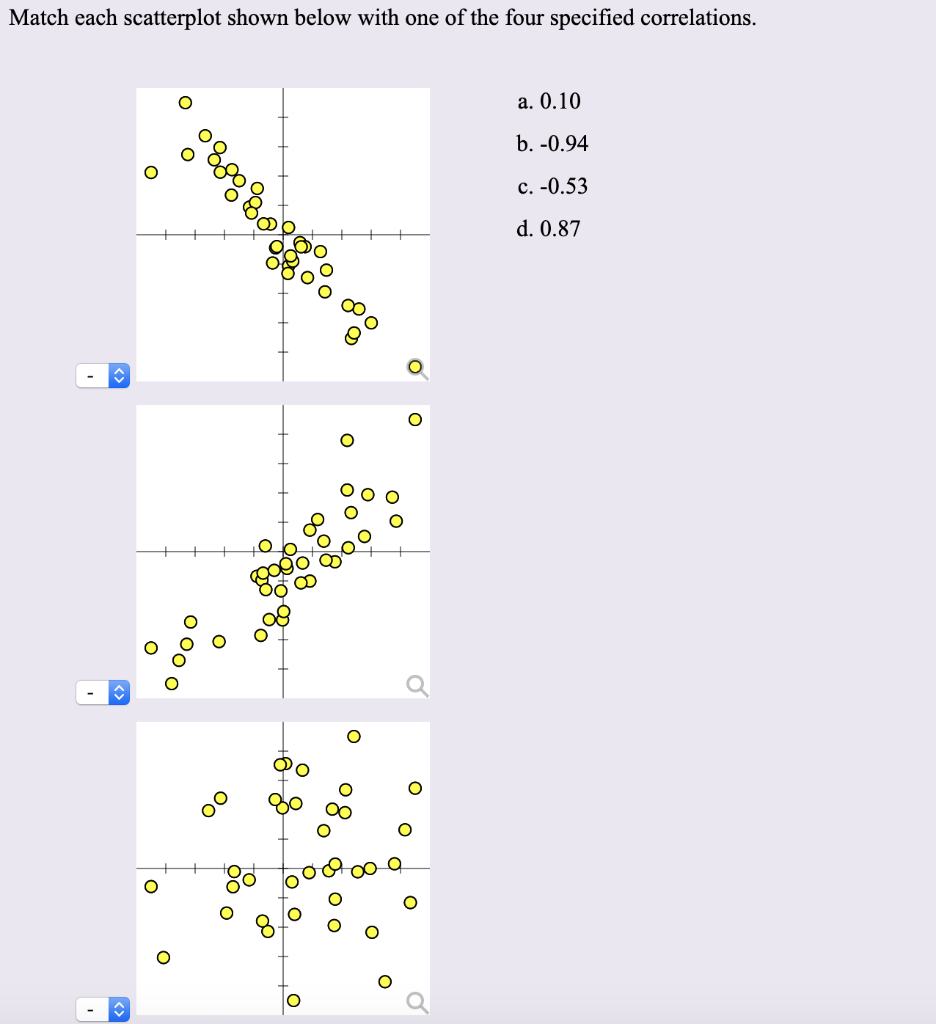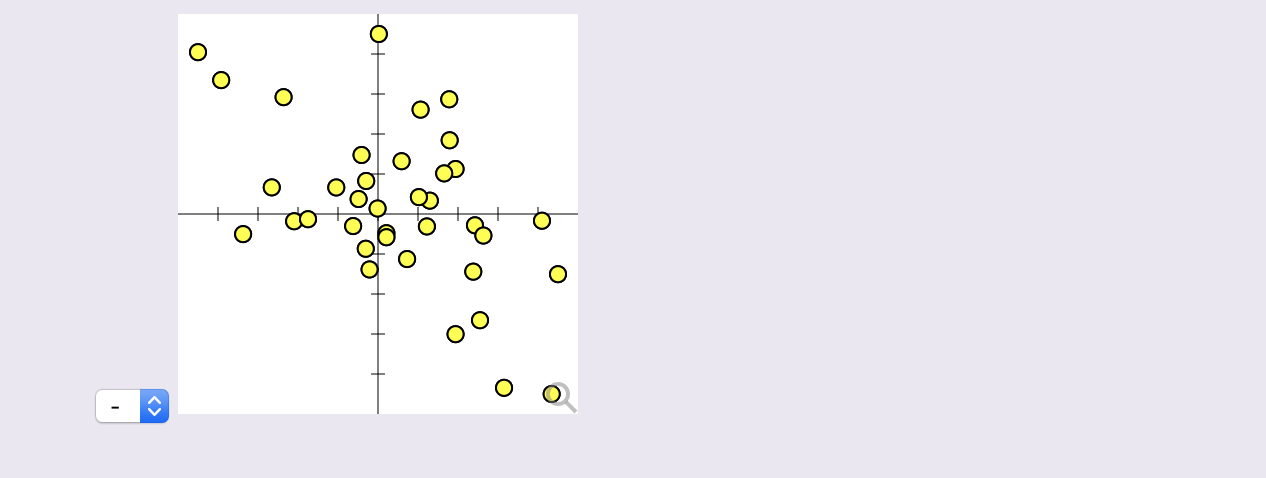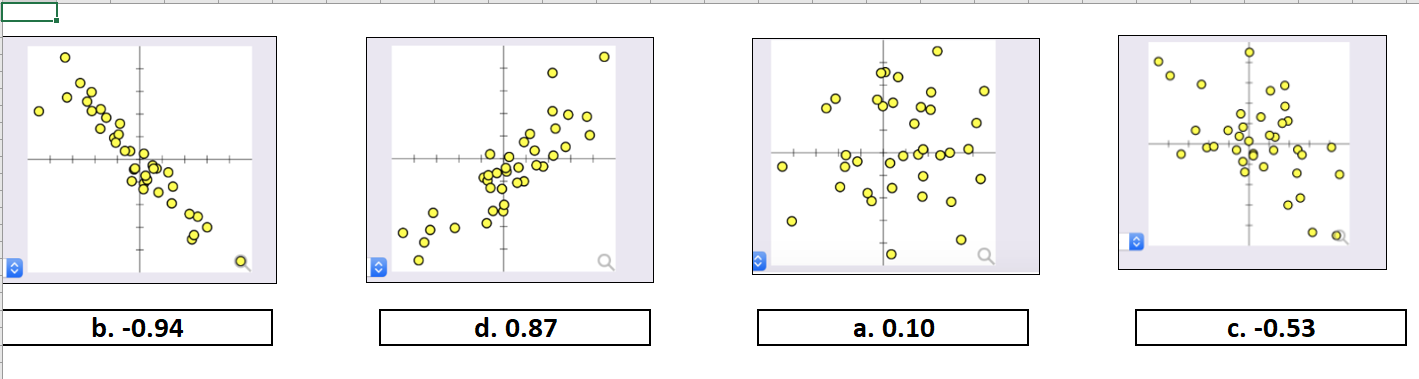We see the direction and the spread of the scatterplot in order to decide the correlation.

If the points are really close to each other then the magnitude of the correlation is high. If the points are scattered then the magnitude of the correlation is low. Then we see the direction, whether Y increases with X (+ve correlation) or decreased(-ve correlation)

Let me know in the comments if anything is not clear. I will reply ASAP! Please do upvote if satisfied!

Graph - 1 :  b. - 0.94 (highly negatively correlated)

Graph - 2 : d. 0.87 (quite positively correlated)

Graph - 3 : a. 0.10 ( thinly positively correlated)

Graph - 4 : c. - 0.53 (medium negatively correlated)

#### Earn Coins

Coins can be redeemed for fabulous gifts.

Similar Homework Help Questions
• ### Match each scatterplot shown below with one of the four specified correlations. a. -0.95 O b....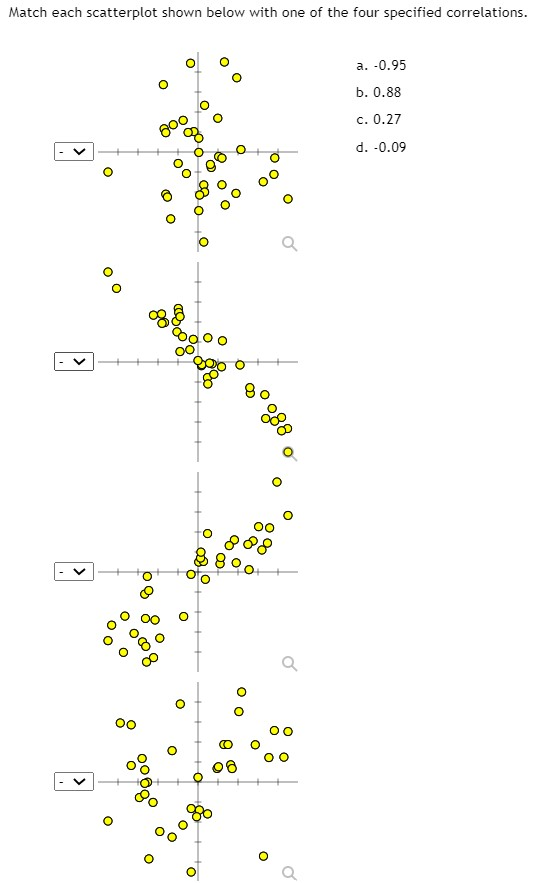Match each scatterplot shown below with one of the four specified correlations. a. -0.95 O b. 0.88 c. 0.27 d. -0.09 < O oo Q og 808 8o O po O 800 O CO o OO B000 o o

• ### Match each scatterplot shown below with one of the four specified correlations. a. -0.90 B b....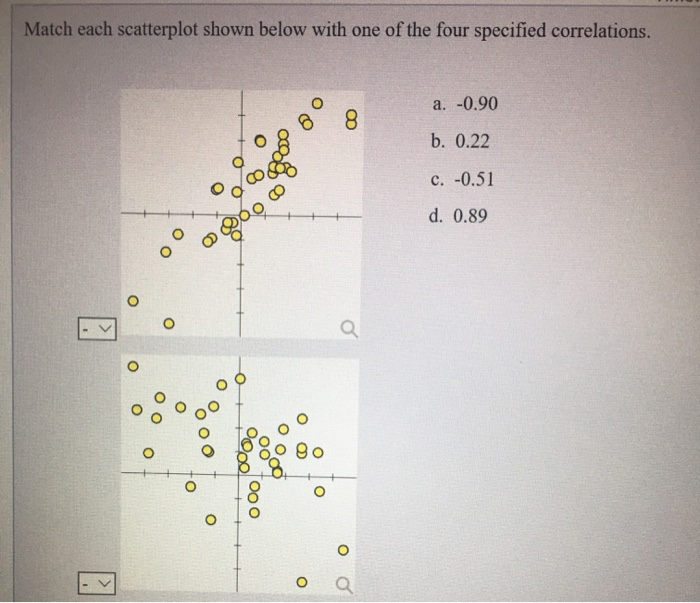Match each scatterplot shown below with one of the four specified correlations. a. -0.90 B b. 0.22 c. -0.51 00 O Xooº 0 0 200 000 d. 0.89 0 tooo yo o 000 000 Oo Oo - 800 ooo • Oo అం ooo 00 10088 to 0 0

• ### Match each scatterplot shown below with one of the four specified correlations a. -0.21 b. 0.99...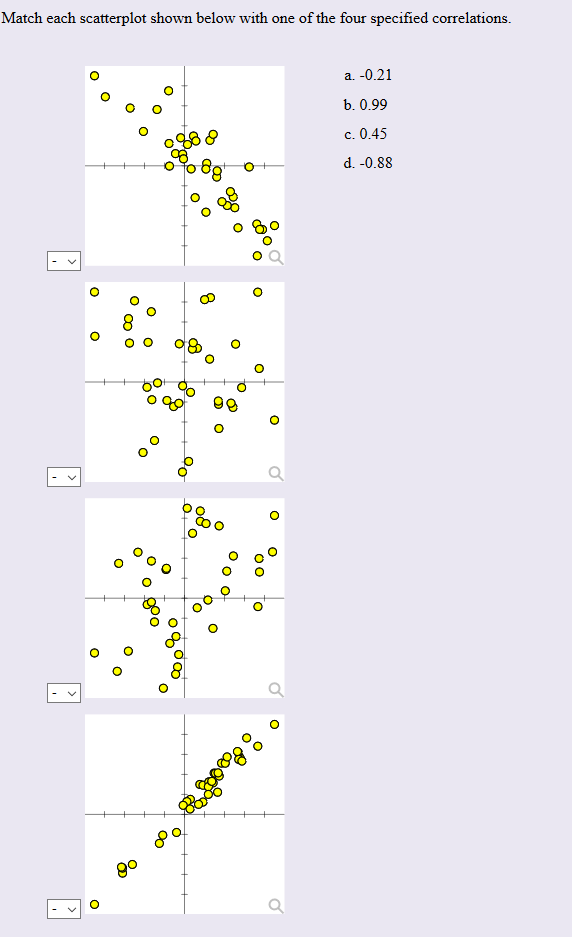Match each scatterplot shown below with one of the four specified correlations a. -0.21 b. 0.99 c. 0.45 d. -0.88 go O O O C o o O o8o O C O

• ### Match each scatterplot shown below with one of the four specified correlations. a.-1.00 b. 0.20 c....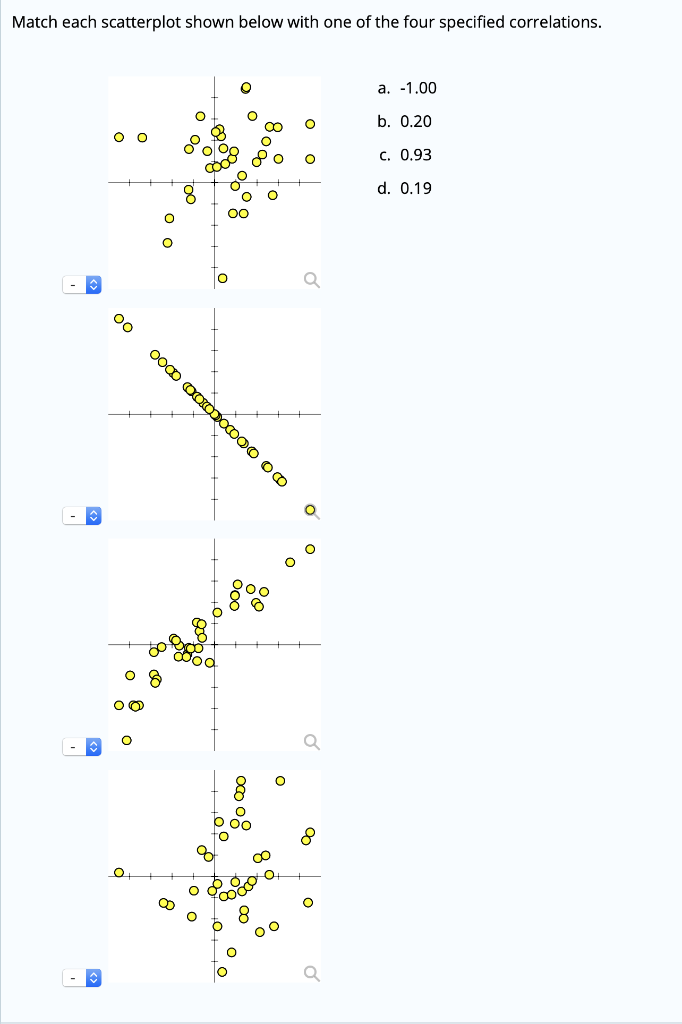Match each scatterplot shown below with one of the four specified correlations. a.-1.00 b. 0.20 c. 0.93 d. 0.19

• ### Match each scatter plot shown below with one of the four specified correlation coefficients. b. 0.25...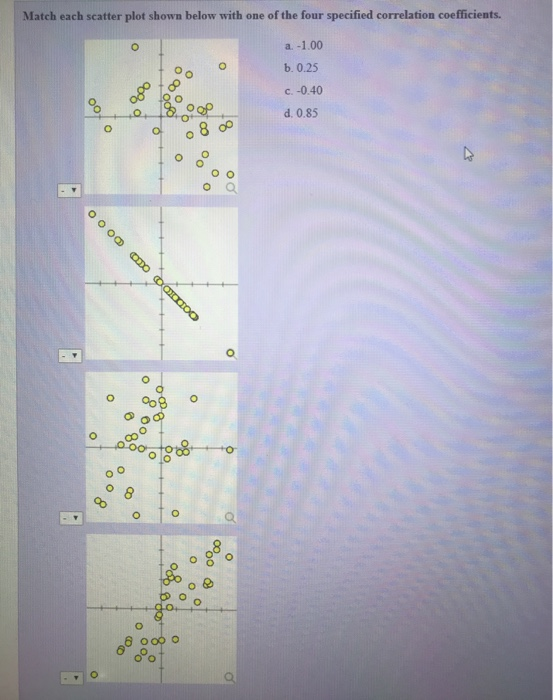Match each scatter plot shown below with one of the four specified correlation coefficients. b. 0.25 c.-0.40 d. 0.85 o% o Q o Oo O lo

• ### Match each scatter plot shown below with one of the four specified correlation coefficients. a 0.97...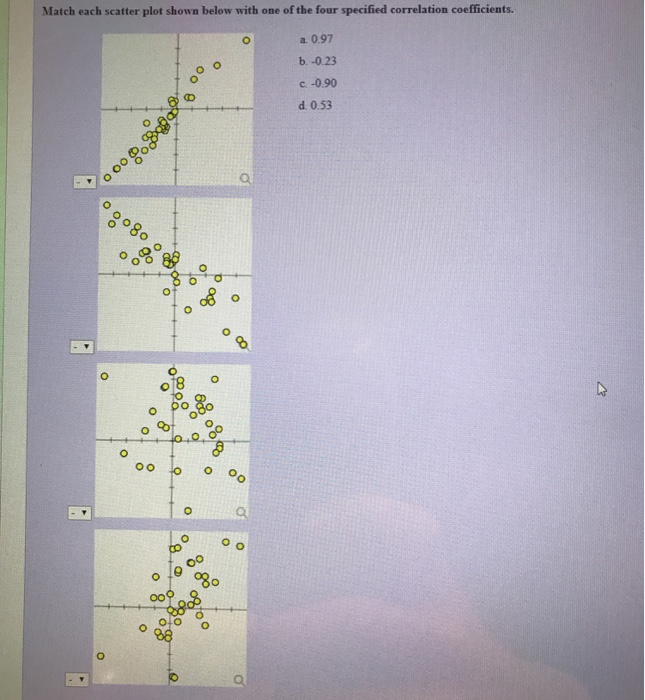Match each scatter plot shown below with one of the four specified correlation coefficients. a 0.97 b.-0.23 C.-090 d 0.53 o18 o o O ob o l9 olo o

• ### Match each scatter plot shown below with one of the four specified correlation coefficients. a-1.00 b.0.25...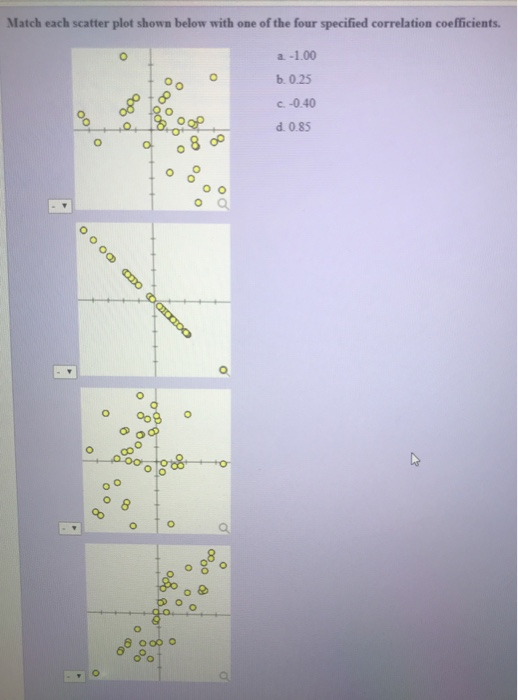Match each scatter plot shown below with one of the four specified correlation coefficients. a-1.00 b.0.25 c. -0.40 d. 0.85 0

• ### Question 4 > You run a regression analysis on a bivariate set of data (n =...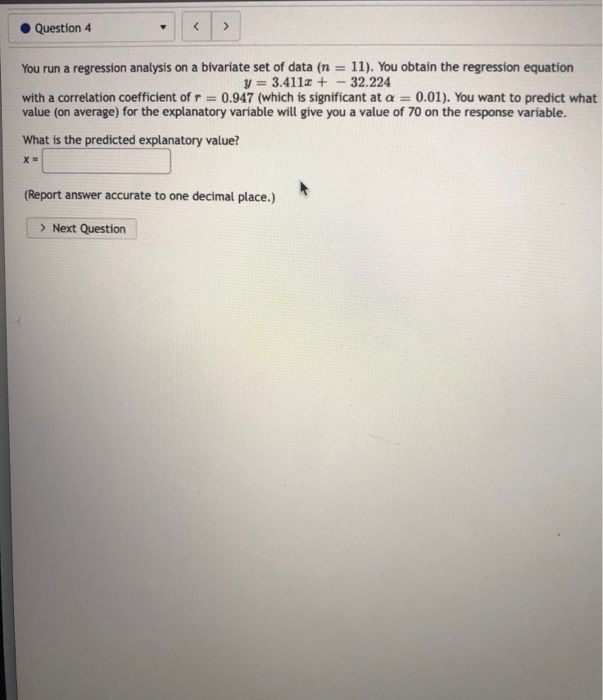Question 4 > You run a regression analysis on a bivariate set of data (n = 11). You obtain the regression equation y = 3.4118 + - 32.224 with a correlation coefficient of r = 0.947 (which is significant at a = 0.01). You want to predict what value (on average) for the explanatory variable will give you a value of 70 on the response variable. What is the predicted explanatory value? X (Report answer accurate to one decimal place.)...

• ### Which one of the four scatterplots below has a correlation coefficient of 0.99? А B ....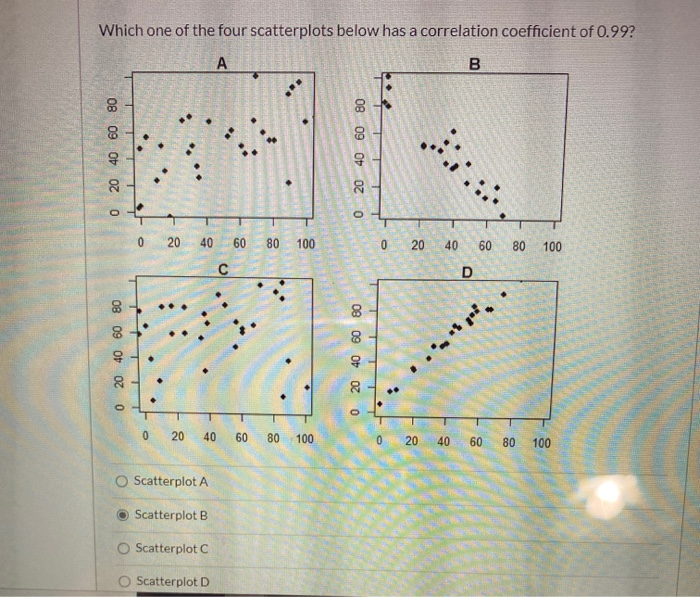Which one of the four scatterplots below has a correlation coefficient of 0.99? А B . 20 40 60 80 0 20 40 60 80 0 0 20 40 60 80 100 0 20 40 60 80 100 с D 0 20 40 60 80 0 20 40 60 80 0 20 40 60 80 100 0 20 40 60 80 100 Scatterplot A Scatterplot B O Scatterplot C Scatterplot D

• ### X A Correlation Cox R Variance calcul x 10.1 Homeworx Connect Maryl X M "Range-brer XM...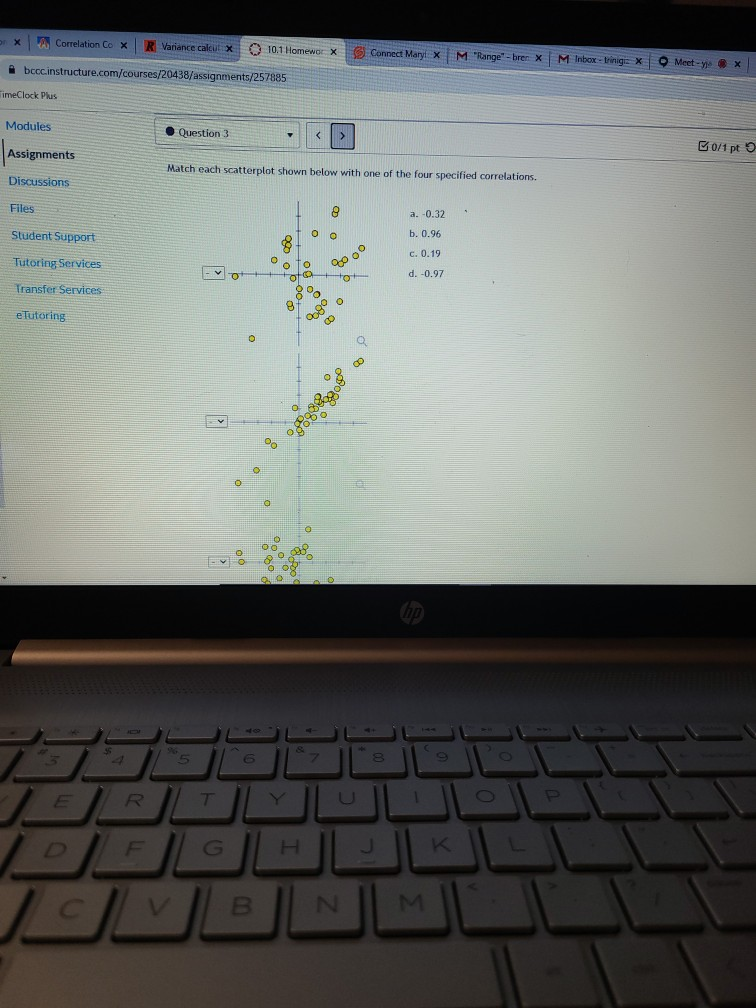X A Correlation Cox R Variance calcul x 10.1 Homeworx Connect Maryl X M "Range-brer XM Inbox -tringe X Meet-X bcccinstructure.com/courses/20438/assignments/257885 TimeClock Plus Modules Question 3 B0/1 pt o Assignments Match each scatterplot shown below with one of the four specified correlations. Discussions Files 8 a. -0.32 Student Support 0 0 b. 0.96 C. 0.19 Tutoring Services oo gooº -ot d. -0.97 Transfer Services oto 8 o e Tutoring 0.8° O 06 o a 8 T DF Н J. K...